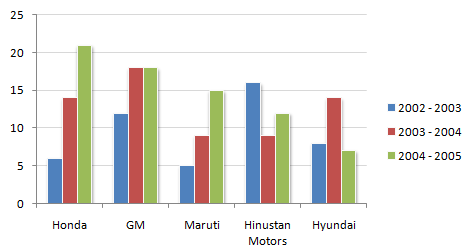# Data Interpretation - Bar Charts

The following chart shows the production of cars in thousands.

Production of Cars for 2002 - 2005 period from the Selected Manufacturers1.

How many companies have shown production below their average production in 2002 - 2003, but have showed above the average production in 2003 - 2004 ?

 A. One B. Two C. Three D. Four

Explanation:

Average sales of company:

Honda = (6 + 14 + 21)/3 = 13.66

GM = (12 + 18 + 18)/3 = 16

Maruti = (5 + 9 + 15)/3 = 9.66

Hindustan Motors= (16 + 9 + 12)/3 = 12.33

Hyundai = (8 + 14 + 7)/3 = 9.66

2.

The ratio of Hindustan Motors production in 2003 - 2004 to Honda's production in 2002 - 2003 is ?

 A. 0.66 B. 1.5 C. 2 D. None of these

Explanation:

The required ratio is (9/6) = 1.5.

3.

For how many companies has there been no decrease in production in any year from the previous year ?

 A. One B. Two C. Three D. Four